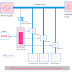## What is an Automatic Power Factor Controller?

The automatic power factor controller is a device that can correct, improve or maintain the power factor level near to unity automatically. In short, it is known as APFC.

When the connected inductive load in the power circuit causes to decrease in the power factor the APFC detects and improves the power factor. We know that the power factor not near to unity can increase the consumption of reactive power. This high reactive power consumption can cause high current flow in the circuit. And this high current flow causes to produce heat, loss, etc. So the power factor more lagging or more leading is harmful to the electrical circuit. Generally, inductive loads are mostly used in our applications that cause lagging power factors. So here we need a capacitive load to maintain the power factor. Basically, the APFC has capacitor banks to improve the power factor.

## Automatic Power Factor Controller(APFC) Block Diagram

Here, you can see the block diagram of automatic power factor controller. It will help a lot to understand the working principle of APFC.

In a practical circuit or device, there are so many circuits, components are available. But here, we have shown the main important parts that are enough to understand its working principle.

## APFC Controller Parts or Components

The main parts of the APFC are,

### Capacitor Bank

It is the main part of the APFC controller. Actually, it only improves the power factor. Inductive load causes a lagging power factor in the electrical circuit but the capacitor bank causes the leading power factor so the power factor can be improved. The capacitor bank is basically a combination of capacitors it has so many capacitors that are internally connected either in delta connection or in start connection. But in most of the cases, they are connected in a delta configuration. The capacitor banks are internally protected by the different types of protective equipment such as fuses, circuit breakers, etc. You can see in the above block diagram we have shown only three capacitor banks but in actuality there are so many capacitor banks are used. According to the requirement to maintain power factor the APFC circuit automatically connects or disconnects the capacitor banks to the main circuit.

### Thyristor

A thyristor is basically an electronic switch. It is connected between the capacitor bank and the main circuit or power line. So when the microcontroller provides a signal or pulse to these thyristors they connect or disconnect the capacitor banks to the main power line. In most of the cases, the TRIAC is used because it is capable to work with AC supply. So the thyristor is operated according to the signals from the microcontroller.

### Microcontroller

The microcontroller is the brain of the whole APFC controller, it controls everything. It analyzes the power factor and decides how much capacitor bank is to be connected to the power line to improve the power factor. According the current power factor level it sends the signal to the thyristor to connect or disconnect the capacitor banks to the power line.

### Power Factor Sensor/Transducer

It is the combination of current transformer or current sensor and power factor sensor. Basically, it works as a medium between the microcontroller and power line for measuring the power factor. The microcontroller measures the current, voltage, and power factor using this power factor sensor or power factor transducer.

## How Automatic Power Factor Controller Works?

The working principle of the Automatic Power Factor Controller is very simple. The microcontroller continuously measures the power factor of the power line. When the power factor decreased. So it will send the signal to the thyristor to connect the capacitor bank to the power line. Again when the power factor increased to more than its requirements, the microcontroller sends the signal to the thyristor to disconnect the capacitor bank. For example, when the power factor is 0.8 lagging the capacitor bank will be activated but when the power factor is 0.7 lagging, then both of the capacitor bank 1 and capacitor bank 2 will be activated. So, now you can understand that more lagging power factor means more capacitor bank activation.

What is Automatic Power Factor Controller? Block DiagramReviewed by Author on February 05, 2022 Rating: 5